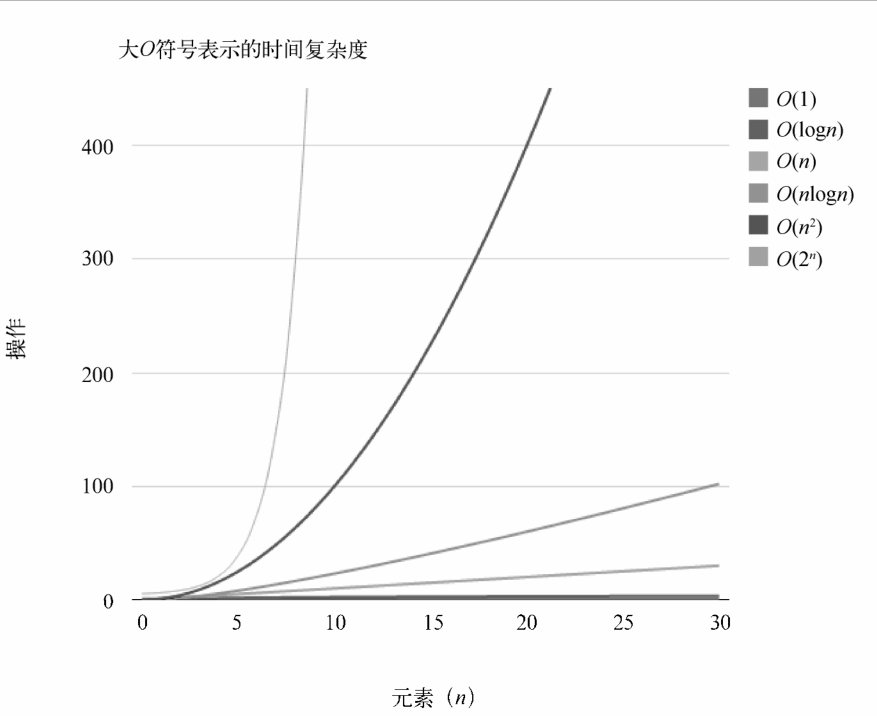## 第十二章 算法复杂度

### 大O表示法

O(1) 常数的
O(log(n)) 对数的
O((log(n)c)) 对数多项式的
O(n) 线性的
O(n2) 二次的
O(nc) 多项式的
O(cn) 指数的

### 时间复杂度比较AVL树 O(log(n)) O(log(n)) O(log(n)) O(log(n)) O(log(n)) O(log(n))

0%